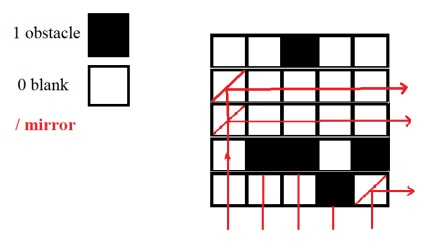# Maximum mirrors which can transfer light from bottom to right in C++

We are given with a square matrix which contains 0’s and 1’s only. 0 represents a blank or empty place and 1 means obstacle. We must find a number of mirrors that can be placed at empty cells such that these mirrors can transfer light from bottom to right. This is possible when, mirror is placed at index [i,j] and for all cells on the right in that particular row ( i ) and cells in the bottom ( j ) in that particular column have no obstacle.

If the mirror is at A[i][j], then all A[i+1 to n][ j ] and A[ i ][ j+1 to n ] are empty i.e, 0. As shown in below figure.## Input

Arr[][] = {{0,0,1,0,0},{0,0,0,0,0},{0,0,0,0,0},{0,1,1,0,1},{1,1,1,0,1}}

## Output

No. of mirrors : 3

Explanation − As shown in figure. Mirrors can be placed at cells

Arr − row 1 and column 0 has all 0’s below and right of it.

Arr − row 2 and column 0 has all 0’s below and right of it.

Arr − Last cell, is 0 and no rows below and column on right.

## Approach used in the below program is as follows

• Array Arr[][] is used to represent matrix of 0’s and 1’s..

• Function maximumMirror(int mat[][], int n) takes matrix and it’s side n as input and returns the count of maximum mirrors that can be placed as shown above.

• Variable flag is used to mark the presence of an obstacle in cells below or cells on the right of arr [i] [j].

• Count is used to represent the number of mirrors, initially 0 .

• Traverse matrix from index 0,0.

• For each cell if it is empty (mirror can be placed), check for cells below( k=i+1 to n-1) . If any arr[k][j] is an obstacle (value=1 ) then break while loop and mark flag as 1. If not, continue checking for cells in right ( l=j+1 to n-1 ).

• Set flag in case an obstacle is present.

• After both while loops if flag is 0 then increment count as mirror can be placed.

• Return count as no. of mirrors that is maximum.

## Example

Live Demo

// C++ program to find how many mirrors can transfer
// light from bottom to right
#include <bits/stdc++.h>
using namespace std;
// method returns number of mirror which can transfer
// light from bottom to right
int maximumMirror(int mat, int N){
// to mark that all cells in the right or bottom are 0---no obstacle
int flag=0;
int count=0; //count of mirrors
int i,j,k,l;
//for all cells
for (int i=0; i<N; i++)
for(j=0;j<N;j++){
//check from next column and next row
int k=i+1;
int l=j+1;
if(mat[i][j]==0) //position for mirror{
while(k<N) //check for rows below{
if(mat[k][j]==1) //keeping column fixed, if there is obstacle break{
flag=0; break; }
else
flag=1;
k++;
}
if(flag==1) //if no obstacle in rows then check columns in right
while(l<N) //checking for columns in right{
if(mat[i][l]==1) //keep row fixed, if obstacle break{
flag=0; break;
}
else
flag=1;
l++;
}
if(flag==1) //there is no obstacle for mirror mat[i][j]
count++;
}
}
return count;
}
int main(){
int N = 5;
//matrix where 1 represents obstacle form 5X5 matrix
int mat = {{0,0,1,0,0},{0,0,0,0,0},{0,0,0,0,0},{0,1,1,0,1},{1,1,1,0,1}};
cout <<"Maximum mirrors which can transfer light from bottom to right :"<<
maximumMirror(mat, N) << endl;
return 0;
}

## Output

Maximum mirrors which can transfer light from bottom to right :3